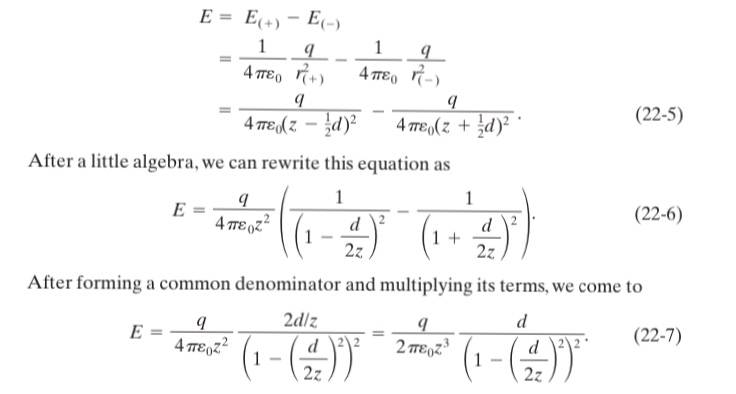# Finding a Common Denominator

• I
• rtareen
rtareen
TL;DR Summary
I have 2 equations, one directly coming from the first through algebra. I dont know how to get from Equation 22-6 to Equation 22-7.I am trying to derive the equation for the field generated by an electric dipole for at a point along the dipole axis. d is the distance between the between the point charges and z is the distance from the center point of the dipole to the point of interest along the dipole axis. I just want to know how you get from equation 22-6 to equation 22-7. Please show all steps.

PS: This assumes that the point is along the side of the axis that is closer to the positive charge. Will it be the same if the point was on the opposite side?

#### Attachments

Mentor
As they wrote, form a common denominator. ##\frac{1}{a}-\frac{1}{b} = \frac{b}{ab}-\frac{a}{ab} = \frac{b-a}{ab}##. Plug in the denominators for a and b, then simplify. To simplify the denominator it helps that ##(x-y)(x+y) = x^2-y^2##

Which step is unclear?

rtareen
As they wrote, form a common denominator. ##\frac{1}{a}-\frac{1}{b} = \frac{b}{ab}-\frac{a}{ab} = \frac{b-a}{ab}##. Plug in the denominators for a and b, then simplify. To simplify the denominator it helps that ##(x-y)(x+y) = x^2-y^2##

Which step is unclear?
Thanks for showing me how they did it. Its been so long since I've used that, I forgot it existed.